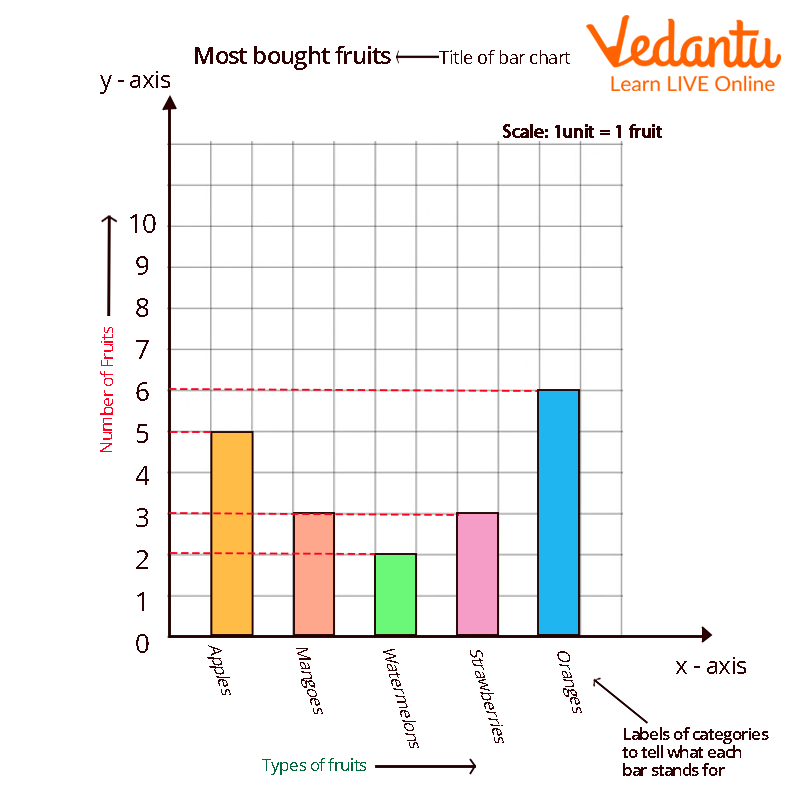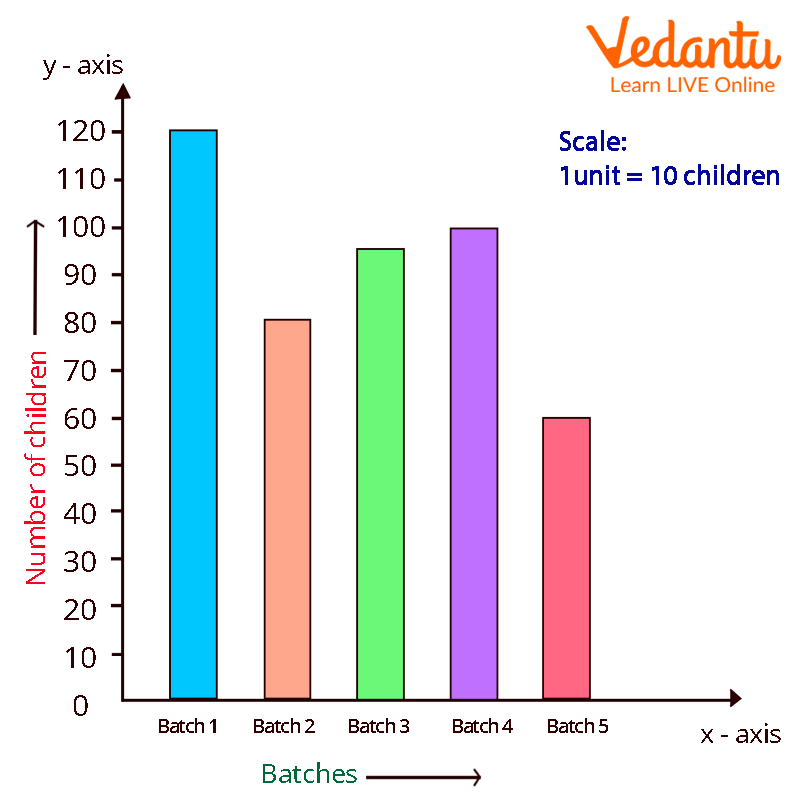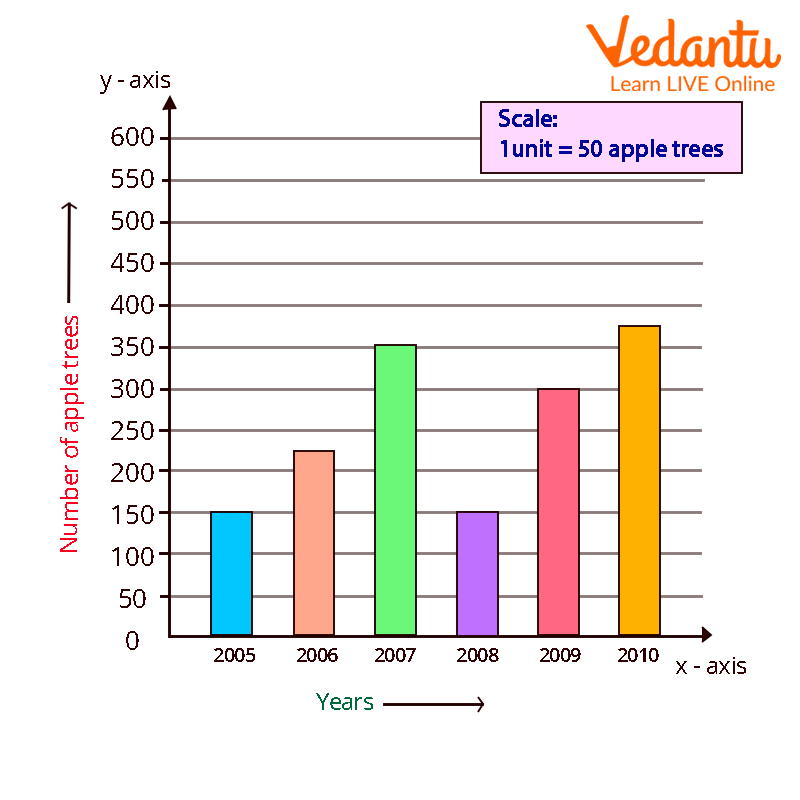Courses
Courses for Kids
Free study material
Offline Centres
More

# Bar Graph for KidsLast updated date: 03rd Dec 2023
Total views: 100.8k
Views today: 1.00k## Bar Graph Definition

A bar graph is a great tool for representing data that are unrelated to one another and do not need to be displayed in any particular sequence. The bars provide a visual representation for comparing amounts in several categories. The x and y axes, commonly known as the horizontal and vertical axes, the title, labels, and a bar graph are all included.

Bar Graph shows all the data, the heights of the rectangular bars are proportional to the values they represent. The graph's bars can be displayed either vertically or horizontally. Bar graphs, commonly referred to as bar charts, are a visual depiction of groups of data. It is one method of processing data.

### What is a Bar Diagram and How to Draw a Bar Graph?

With the help of an example, let's learn how to draw a bar graph. Lana visited the market to purchase a variety of fruits, including 5 apples, 3 mangoes, 2 watermelons, 3 strawberries, and 6 oranges. She wants to create a bar graph to present the data so she can see what kinds of fruits she purchases the most frequently.

Let's use the next few stages to create a bar graph of the fruit that is most frequently purchased.

Step 1: Take a piece of graph paper, and label the bars with a caption like "Most Bought Fruit."

Step 2: On a plane, draw the horizontal (x-axis) and vertical (y-axis) axes.

Step 3: Give the horizontal axis, which is an independent category, the name "Types of Fruits," and the vertical axis, which is a dependent category, the name "Number of Fruits."

Step 4: Write the names of the fruits on the horizontal axis, such as apples, mangoes, watermelon, strawberries, and oranges, and leave an equal gap or spacing between each fruit.

Step 5: Describe the graph's scale, which demonstrates how numbers are used in the data. It is a system of markers spaced at specific intervals that aids in object measurement.

Step 6: Start constructing rectangular bars for each fruit, leaving equal spaces between them, and assigning heights to the corresponding numbers.

Step 7: Now that the bar graph is complete, determine which fruit is the most popular by measuring the height of the rectangle bars for each fruit.Bar Diagram Example

It is crucial to note four elements while creating a bar graph: the title, scale, and names of the axes.

## Bar Graphs Parts

There are two basic categories for bar graphs:

• Vertical Bar Graph

• Horizontal Bar GraphBar Graph Parts

## Properties of Bar Graph

The following list includes some characteristics that set a bar graph apart from other types of graphs:

• The width and spacing between each rectangular bar should be the same.

• Either horizontally or vertically can be used to draw the rectangular bars.

• The rectangular bars' height is equal to the height of the data they depict.

• There must be a common base for the rectangular bars.

## Conclusion

A bar graph is a great method for showing data that does not need to be displayed in any specific order and is unconnected to one another. The bars offer a visual way to compare quantities across many categories. The title, labels, x and y axes, also known as the horizontal and vertical axes, as well as a bar graph are all present.

## Solved Examples

Example 1: The following information about a school's five separate batches of students. Create a bar graph to represent the following data.

 BATCHES NUMBER OF STUDENTS Batch 1 120 Batch 2 80 Batch 3 95 Batch 4 100 Batch 5 60

Answer: The data can be represented as:Example 2: What kinds of bar graphs are there?

Answer: Vertical bar graphs, horizontal bar graphs, stacked bar graphs, and grouped bar graphs are the four different types of bar graphs. A vertical bar graph shows the grouped data in a vertical direction. A horizontal bar graph shows the grouped data in a horizontal direction.

Example 3: The number of apple trees a school's gardener planted over the course of many years is displayed in the following table. Create a bar graph to represent the below given data.

 YEARS NUMBER OF APPLE TREES 2005 150 2006 220 2007 350 2008 150 2009 300 2010 380

Answer: Above data can be represented as:## FAQs on Bar Graph for Kids

1. Mention any 2 uses of bar graph.

1. It is the most popular technique for representing data. As a result, it is utilized by numerous industries.

2. Data sets are compared using it.

2. What are the purposes of bar charts?

When displaying the distribution of data points or comparing metric values across several subgroups of your data, a bar chart is employed.

3. What distinguishes a pie chart from a bar graph?

In contrast to the pie chart, which is a sort of graph in which a circle is divided into parts and each portion is a fraction of the whole, the bar chart displays discrete data while comparing one data point with another.

4. What does the bar graph's title say?

The bar graph's title makes clear what the graph is about. It depicts the data that we ultimately extrapolate from the graph supplied.